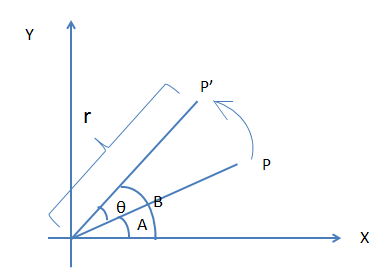# 变换矩阵

$\begin{bmatrix} a & b & 0 \\ c & d & 0 \\ t_{x} & t_{y} & 1 \end{bmatrix}$

Apple采用了用补齐1x3的向量，和用[0,0,1]的转置补齐的3x3的变换矩阵相乘来做仿射变换。虽然可能觉理论上可以直接用2x3变换矩阵和3x1的向量([x,y,1]的转置)运算，得到一个2x1的向量，省3个CGFloat的空间。但是由于这种变换操作叠加次数特别多，与其每次得到的向量结果再补齐，还不如一次性就用一个1x3和3x3运算，用空间换取时间，这也许是QuartzCore的实现者的考虑吧。

$\begin{bmatrix} x & y & 1 \end{bmatrix} \times \begin{bmatrix} a & b & 0 \\ c & d & 0 \\ t_{x} & t_{y} & 1 \end{bmatrix} = \begin{bmatrix} ax+cy+t_{x} & bx+dy+t_{y} & 1 \end{bmatrix}$

# 平移

$\begin{bmatrix} x & y & 1 \end{bmatrix} \times \begin{bmatrix} 1 & 0 & 0 \\ 0 & 1 & 0 \\ a & b & 1 \end{bmatrix} = \begin{bmatrix} x+a & y+b & 1 \end{bmatrix}$

# 缩放

$\begin{bmatrix} x & y & 1 \end{bmatrix} \times \begin{bmatrix} a & 0 & 0 \\ 0 & a & 0 \\ 0 & 0 & 1 \end{bmatrix} = \begin{bmatrix} ax & by & 1 \end{bmatrix}$

# 旋转

$\begin{bmatrix} x & y & 1 \end{bmatrix} \times \begin{bmatrix} \cos \theta & \sin \theta & 0 \\ - \sin \theta & \cos \theta & 0 \\ 0 & 0 & 1 \end{bmatrix} = \begin{bmatrix} x \cos \theta - y \sin \theta & x \sin \theta + y \cos \theta & 1 \end{bmatrix}$$P = (x,y) = (r\cos A , r\sin A) \\ P^{‘} = (r \cos B, r \sin B) = (r\cos(A + \theta), r\sin(A + \theta)) \\ r\cos(A + \theta) = r\cos A \cos \theta - r\sin A \sin \theta = x \cos \theta - y \sin \theta \\ r\sin(A + \theta) = r\sin A \cos \theta + r\cos A \sin \theta = y \cos \theta + x \sin \theta \\ \therefore M = \begin{bmatrix} \cos \theta & \sin \theta & 0 \\ - \sin \theta & \cos \theta & 0 \\ 0 & 0 & 1 \end{bmatrix}$

# 错切$\begin{bmatrix} x & y & 1 \end{bmatrix} \times \begin{bmatrix} 1 & 0 & 0 \\ m & 1 & 0 \\ 0 & 0 & 1 \end{bmatrix} = \begin{bmatrix} x+my & y & 1 \end{bmatrix}$

# 参考资料

iOS Core Animation Advanced Techniques